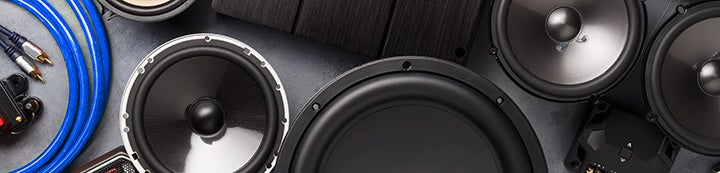1 - 1 of 1 Posts

#### Audio_phill

·
##### Walking TSP Encyclopedia
Joined
·
2,300 Posts
Discussion Starter · ·
SW8000 (full break-in)

Re = 3.0347 ohms
Fs = 24.4238 Hz
Zmax = 24.9541 ohms
Qes = 0.6189
Qms = 4.4707
Qts = 0.5437
Le = 1.0843 mH (at 1 kHz)
Diam = 209.5500 mm ( 8.2500 in )
Sd =34487.7765 mm^2( 53.4562 in^2)
Vas = 40.6835 L ( 1.4367 ft^3)
BL = 11.5191 N/A
Mms = 176.3554 g
Cms = 240.7817 uM/N
Kms = 4153.1392 N/M
Rms = 6.0535 R mechanical
Efficiency = 0.0900 %
Sensitivity= 81.5596 dB @1W/1m

SW8200 (Some break-in)

Re = 3.2856 ohms
Fs = 22.0150 Hz
Zmax = 23.5699 ohms
Qes = 0.9382
Qms = 5.7920
Qts = 0.8074
Le = 1.0685 mH (at 1 kHz)
Diam = 256.5400 mm ( 10.1000 in )
Sd =51689.2299 mm^2( 80.1185 in^2)
Vas = 87.1135 L ( 3.0764 ft^3)
BL = 10.5028 N/A
Mms = 227.7101 g
Cms = 229.5200 uM/N
Kms = 4356.9180 N/M
Rms = 5.4381 R mechanical
Efficiency = 0.0931 %
Sensitivity= 81.7071 dB @1W/1m

•Vmann
1 - 1 of 1 Posts